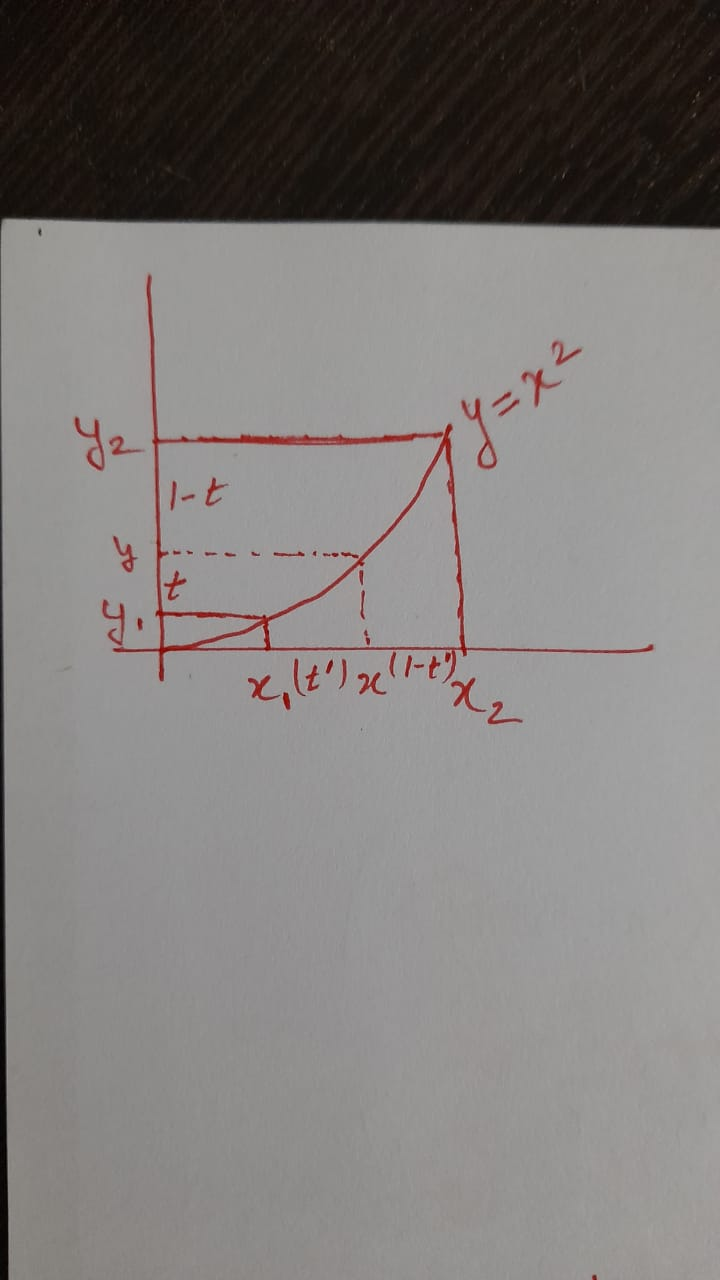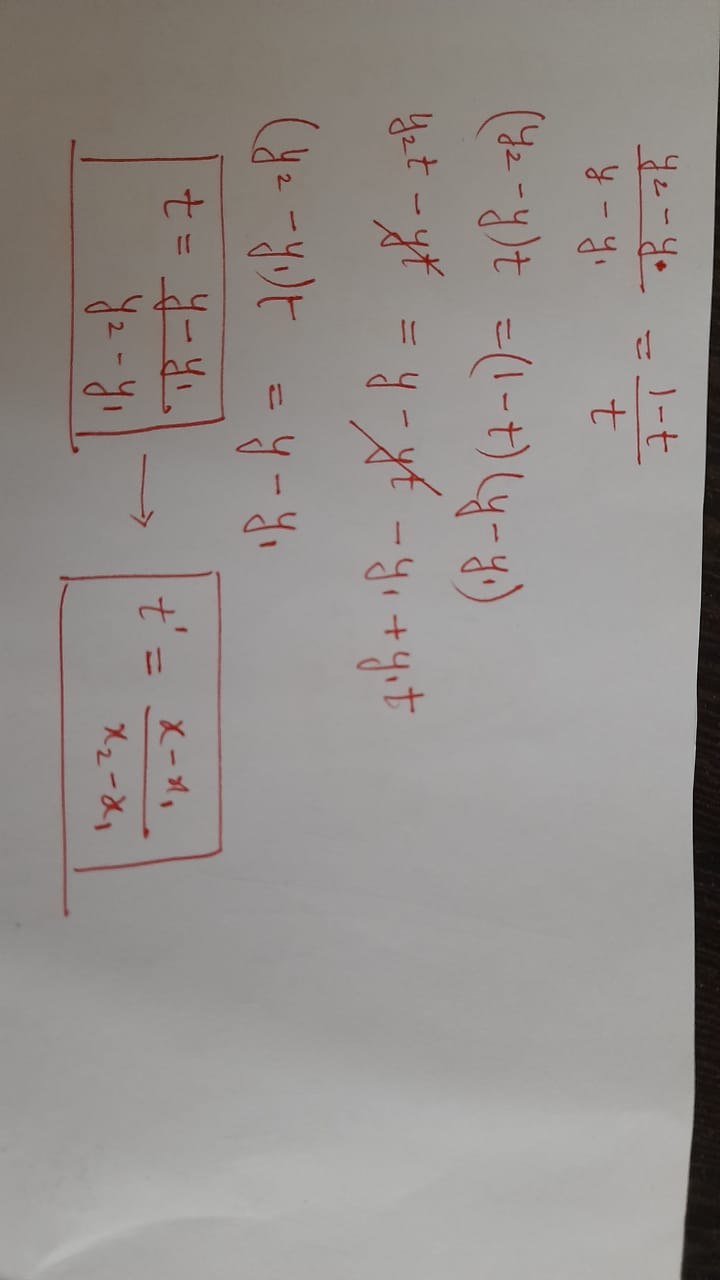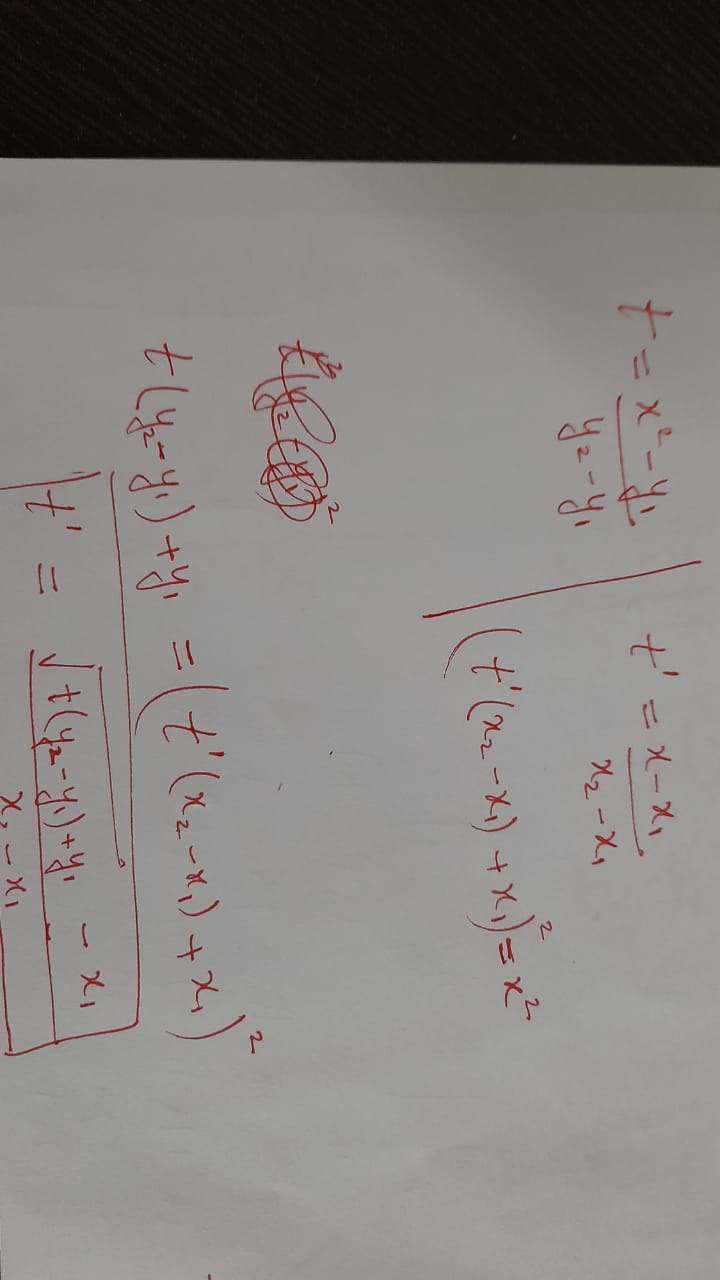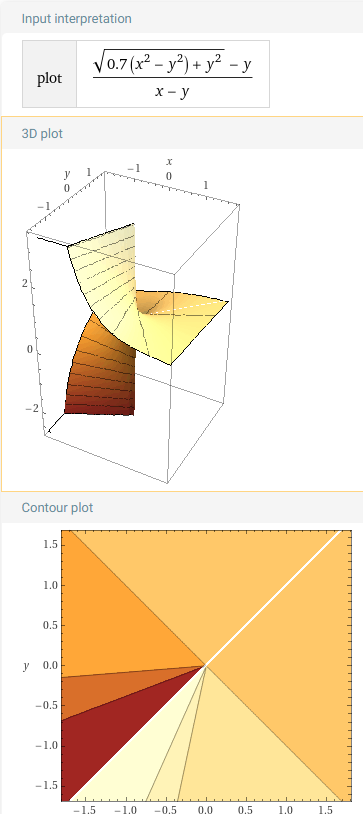Gaurav Gautam
96 posts
Day94: Can we do blending math in the sRGB space instead of candela space?
Edited by Gaurav Gautam on

There was a question in this episode or episode95 where someone asked if we could just do the math of blending directly in RGB space. Casey Muratori noted that the equation for the blend in RGB space looked like the pythagoras theorem but nothing came out of it. I have tried to figure out an equation that seems to allow me to blend directly in the RGB space. Can someone tell me if I'm wrong about this?

So assuming the y=x^2 curve for gamma I have obtained that if you want to blend two values say (y1 and y2) with a ratio of t in the candela space (y-axis), then you can instead blend the corresponding x1 and x2 values by the ratio tprime given by

tprime = (sqrt(t*(y2-y1) + y1) - x1)/(x2 - x1)


This still has a square root so it will be no more efficient than just blending in candela space, but you really can just blend in rgb space.

Here is my derivation for the above expression:

1. We take the x1, x2 and corresponding y1 and y2 values on the y=x^2 curve as follows. We assume we are trying to blend the y1 and y2 by the ratio t and 1-t as shown on y axis. Furthermore, we note the corresponding ratio of tprime on the xaxis1. Next we get the expression of t as a fraction between the y, y1 and y2 values. And also the same kind of expression between tprime, x, x1 and x2 shown in boxes below.1. Now since we know that y is always x^2 we can eliminate the x from these equations as follows:Since we know x1, x2 and t, and y1 and y2 are just x1^2 and x2^2, we can find the tprime for any given t, x1 and x2. And once we know that the blend can be done as x1*(1-tprime) + x2*tprime.

This exercise doesn't give us any computational advantage though since there is still a root to be calculated for each of the r,g and b values. However, maybe it reduces the code complexity a bit by allowing us to just work with the rgb values all the time? That claim is a bit stretched really. But, am I right in saying that we indeed can do the interpolation directly in rgb space like this at least in the approximation of x^2?

P.S. I have not tried to graph the values of tprime vs t for different values of x1 and x2. It looks like it will be some kind of weird surface. But, if that surface has some flat parts in the square of (0,0) to (1, 1) I wonder if we can pull some trick where we don't have to compute all the tprimes for all the x1,x2,t's. Maybe we can do some kind of lookup table (although this is not discrete space) to optimize on the number of computations we have to do?

Gaurav Gautam
96 posts
Day94: Can we do blending math in the sRGB space instead of candela space?

I tried to plot itIt doesn't look like there are any contour lines to exploit...

Mārtiņš Možeiko
2470 posts / 2 projects
Day94: Can we do blending math in the sRGB space instead of candela space?

I don't know about math portion of optimizing this, but on actual GPU often this blending in sRGB space is for free. Because GPU includes dedicated hardware space to do the conversion to/from linear space for blending. You just need to set up your render target/texture formats to sRGB and that's it. Blending will happen correctly, no need to worry about extra operations in shader (as there will be none).

Gaurav Gautam
96 posts
Day94: Can we do blending math in the sRGB space instead of candela space?

I see. I know nothing about the GPU yet. Can't wait to get there in HMH series...

BTW, do you also seed compressed videos (as in downconverted to some lower res) of hmh from your server? Right now I have my harddisk almost half full and I have just have the 590-600+ videos. I'll probably order another 2TB hard disk though so no worries if you don't seed low res videos. Can you tell me what is the current size of the full set right now though?

Mārtiņš Možeiko
2470 posts / 2 projects
Day94: Can we do blending math in the sRGB space instead of candela space?

They are original videos Casey shares from his OBS recording. Downloading them from youtube would give you smaller size (and lower quality) as they recompress videos on upload.

Gaurav Gautam
96 posts
Day94: Can we do blending math in the sRGB space instead of candela space?

Yes in the thread you say:

Now choose "Sync all" to be On or Off. When it is ON all files will be downloaded. Each episode is something like ~900MB. Currently total size for all 349 episodes/chats is 317GB).

So nowsince there are 650+ episodes I assume it would be 700-800GB total, but my harddisk is taking ~400GB already. So I guess either the length or quality of videos increased later in the series. I am ordering a new external hdd, but I wanted to make sure I got enough extra space for all of it.

Mārtiņš Možeiko
2470 posts / 2 projects
Day94: Can we do blending math in the sRGB space instead of candela space?